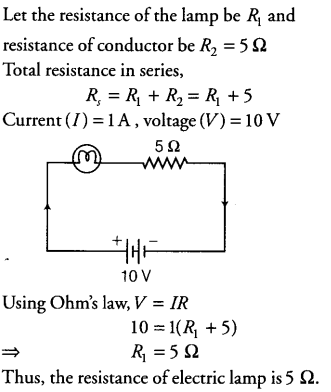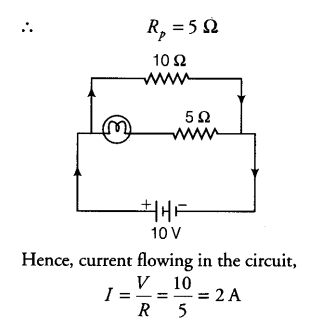# (i) A current of 1 A flows in a series circuit having an electric lamp and a conductor

(i) A current of 1 A flows in a series circuit having an electric lamp and a conductor of 5 \$\Omega \$ when connected to a 10 V battery. Calculate the resistance of the electric lamp.
(ii) Now, if a resistance of 10 \$\Omega \$ is connected in parallel with this series combination, what change (if any) in current flowing through 5 \$\Omega \$ conductor and potential difference across the lamp will take place? Give reason.

(i)(ii) Now, a resistance of 10 \Omega is connected in parallel with the series combination. Therefore, the total resistance of the circuit is given byThus, 1 A current will flow through 10 \Omega resistor and 1 A will flow through the lamp and conductor of 5 \Omega resistance. Hence, there will be no change in current flowing through 5 \Omega conductor. Also, there will be no change in potential difference across the lamp.